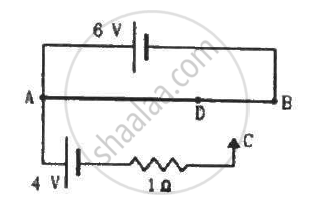Advertisement Remove all ads

# A 6-volt Battery of Negligible Internal Resistance is Connected Across a Uniform Wire Ab of Length 100 Cm. the Positive Terminal of Another Battery of Emf 4 V - Physics

Sum

A 6-volt battery of negligible internal resistance is connected across a uniform wire AB of length 100 cm. The positive terminal of another battery of emf 4 V and internal resistance 1 Ω is joined to the point A, as shown in the figure. Take the potential at B to be zero. (a) What are the potentials at the points A and C? (b) At which point D of the wire AB, the potential is equal to the potential at C? (c) If the points C and D are connected by a wire, what will be the current through it? (d) If the 4 V battery is replaced by a 7.5 V battery, what would be the answers of parts (a) and (b)?Advertisement Remove all ads

#### Solution

(a) Potential difference across AB = Potential at A - Potential at B

Potential at B = 0 V

⇒ Potential at point A = Potential difference across AB = 6 V

⇒ Potential difference across AC = Potential at A - Potential at B

⇒ 4 = 6 - Potential at C

⇒ Potential at C = 2 V

(b) Given:-

Potential across AD = Potential across AC = 4 V

⇒ Potential across DB = 2 V

$\frac{V_{AD}}{V_{BD}} = \frac{l_{AD}}{l_{DB}}$

$\Rightarrow \frac{4}{2} = \frac{l_{AD}}{100 - l_{AD}}$

$\Rightarrow 4\left( 100 - l_{AD} \right) = 2 l_{AD}$

$\Rightarrow 6 l_{AD} = 400$

$\Rightarrow l_{AD} = \frac{400}{6} = 66 . 7 cm$

(c) When the points C and D are connected by a wire, current flowing through the wire will be zero because the points are at the same potential.

(d) Potential difference across AC = Potential at A - Potential at C

⇒ 7.5 = 6 - Potential at C

⇒ Potential at C = −1.5 V

Since the potential at C is negative now, this point will go beyond point B, which is at 0 V. Hence, no such point D will exist between the points A and B.

Concept: Potential Difference and Emf of a Cell
Is there an error in this question or solution?
Advertisement Remove all ads

#### APPEARS IN

HC Verma Class 11, Class 12 Concepts of Physics Vol. 2
Chapter 10 Electric Current in Conductors
Q 55 | Page 202
Advertisement Remove all ads
Advertisement Remove all ads
Share
Notifications

View all notifications

Forgot password?